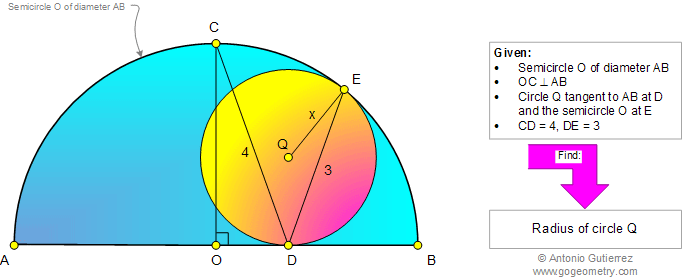# Online Geometry Problem 936: Circle, Semicircle, Diameter, Tangent, Radius, Chord, Metric Relations. Level: High School, SAT Prep, College, Mathematics Education

< PREVIOUS PROBLEM  |  NEXT PROBLEM >

 The figure below shows a semicircle of center O and diameter AB. OC is perpendicular to AB and circle Q is tangent to AB at D and the semicircle O at E. If CD = 4 and DE = 3, find the radius of circle Q.Home | Search | Geometry | Problems | All Problems | Open Problems | Visual Index | 10 Problems | 931-940 | Triangles | Circles | Semicircle | Circle Tangent Line | Tangent Circles | Email | Solution / comment | By Antonio Gutierrez